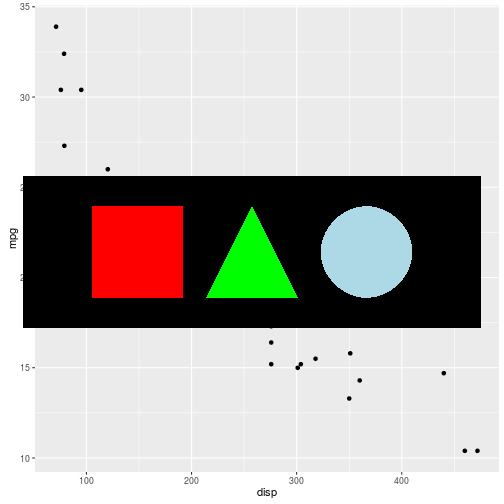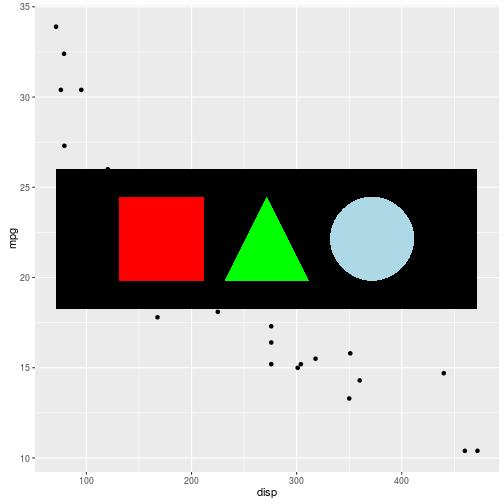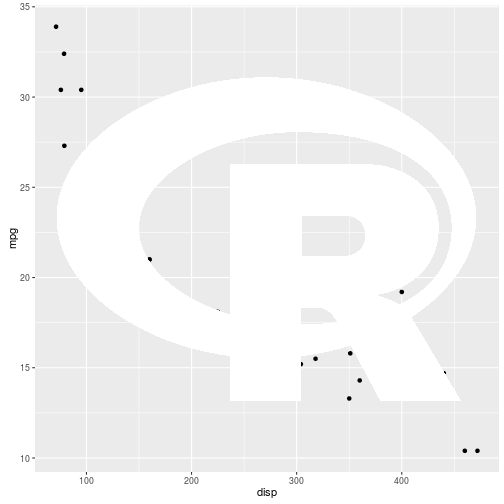Importing the New R Logo

by Paul Murrell

Wednesday 17 February 2016

The R project has a new logo (shown below). On his blog, Bob Rudis showed how to draw the new R logo as part of an R plot. The purpose of this report is demonstrate an alternative way to draw the new R logo in an R plot that makes use of the 'grConvert' and 'grImport2' packages for R.Motivation

The original blog post worked with the SVG format of the R logo (rather than the PNG format), which is nice because it should render well at any scale. The SVG logo was converted to WKT format so that it could then be read into R using the 'rgeos' package, converted to a spatial object using the 'sp' package, then drawn using 'ggplot2'. The result is shown below.One drawback with this result is that the fill colours in this rendering of the logo are flat; the real R logo has a (subtle) gradient fill in both the grey orbit and in the letter R itself.

The colours are flat because the conversion from SVG to WKT only preserved the outline shapes of the logo, not the coloured fills.

An alternative rendering

An alternative approach to rendering the R logo in an R plot involves importing the SVG version of the R logo directly into R. This will allow the gradient fills to be imported into R as well. To do this we need two R packages: 'grConvert' and 'grImport2'.

The 'grImport2' package

The 'grImport2' package for R imports SVG images directly into R. For example, consider the SVG image below ...We can import that into R with the following ...

library(grImport2)

... and render it with the following (using 'ggplot2' just to make it obvious that the result was drawn with R) ...

library(ggplot2)
ggplot(mtcars) +
geom_point(aes(x=disp, y=mpg))
grid.picture(simple)That drawing does not coordinate the imported image with the 'ggplot2' plot; it just draws the image over the top of the plot. We can use the annotation_custom() function to coordinate the image with the plot, but that requires a couple of changes: we need to create a grob from the imported image (using pictureGrob()) rather than just drawing the image (with grid.picture()); and we need to wrap the grob within a gTree because annotation_custom() makes some destructive changes to the grob it is given. The required code is shown below.

library(grid)
simpleGrob <- gTree(children=gList(pictureGrob(simple)))
ggplot(mtcars) +
geom_point(aes(x=disp, y=mpg)) +
annotation_custom(simpleGrob)The 'grConvert' package

A caveat with the 'grImport2' package is that it only supports a subset of the SVG format (because supporting all of SVG is a big job, especially features like font support). The subset of SVG that 'grImport2' supports is the SVG output that is produced by the Cairo graphics library (one reason is that Cairo produces paths for text, rather than using fonts).

R uses the Cairo graphics library for SVG output, so SVG that is drawn with R's svg() device should import fine using 'grImport2'. However, in general, it will be necessary to convert an SVG image to Cairo SVG format. This is what the 'grConvert' package is for.

The following code converts the R logo (in SVG format) to Cairo SVG.

library(grConvert)
convertPicture("Rlogo.svg", "Rlogo-cairo.svg")

Having converted the logo, we can now import it using 'grImport2', as shown below.

RlogoGrob <- gTree(children=gList(pictureGrob(Rlogo)))
ggplot(mtcars) +
geom_point(aes(x=disp, y=mpg)) +
annotation_custom(RlogoGrob)This result is obviously not ideal because, although we have imported the R logo with all of its gradient fill information, the core R graphics system is incapable of rendering gradient fills. So we have one last step to perform.

The 'gridSVG' package

The 'gridSVG' package allows us to render (grid-based) R graphics to an SVG format, with access to SVG features that the core R graphics engine does not support, such as gradient fills.

For example, the following code creates a rectangle with a gradient fill ...

library(gridSVG)
grid.rect(name="r")

... which renders with no fill on a normal R graphics device ...... but which renders with a gradient fill when drawn using the gridsvg() device ...The following code shows that, when we draw an imported image with 'grImport2', we can specify that we want to use 'gridSVG' features in the rendering ...

RlogoSVGgrob <- gTree(children=gList(pictureGrob(Rlogo, ext="gridSVG")))

... and if we then produce SVG output using the gridsvg() device, we get the R logo in all its gradient-filled glory.

gridsvg("Rlogo-ggplot2.svg")
ggplot(mtcars) +
geom_point(aes(x=disp, y=mpg)) +
annotation_custom(RlogoSVGgrob)
dev.off()Summary

If we have an SVG image that we want to include in an R plot, we can do the following:

• Convert the SVG to Cairo SVG with convertPicture() from the 'grConvert' package.
• Import the Cairo SVG with readPicture() from the 'grImport2' pacakge.
• Draw the imported image with grid.picture() from the 'grImport2' package.
• If the imported image contains SVG features that are not supported by the core R graphics engine, use grid.picture(..., ext="gridSVG") and then render on the gridsvg() device from the 'gridSVG' package.

Acknowledgements

The 'grConvert' and 'grImport2' packages were primarily developed by Simon Potter as part of a Google Summer of Code 2013 project.

System requirements

This report was generated on Ubuntu 14.04 64-bit running R 3.2.3 with version 0.1-3 of the 'grImport2' package, version 0.1-0 of the 'grConvert' package, and version 1.5-1 of the 'gridSVG' package. Note that the 'grConvert' package has only been tested on Linux; it has system requirements that may be difficult to satisfy on Windows.

Resources

The raw source file for this report, a valid XML transformation of the source file, a 'knitr' document generated from the XML file, two XSL files that are used to transform the XML to the 'knitr' document, and a Makefile that contains code for the other transformations and coordinates everything.